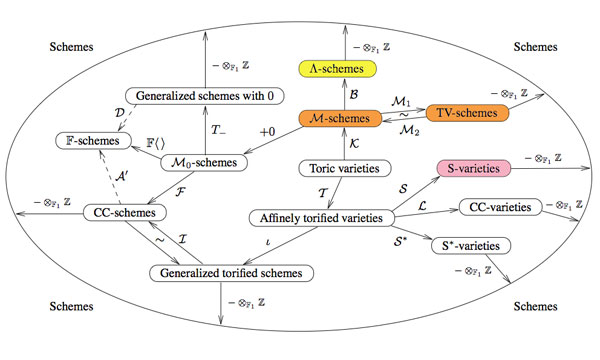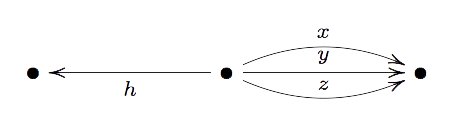Reineke’s observation that any projective variety can be realized as a quiver Grassmannian is bad news: we will have to look at special representations and/or dimension vectors if we want the Grassmannian to have desirable properties. Some people still see a silver lining: it can be used to define a larger class of geometric objects over the elusive field with one element $\mathbb{F}_1$.In a comment to the previous post Markus Reineke recalls motivating discussions with Javier Lopez Pena and Oliver Lorscheid (the guys responsable for the map of $\mathbb{F}_1$-land above) and asks about potential connections with $\mathbb{F}_1$-geometry. In this post I will ellaborate on Javier’s response.

The Kapranov-Smirnov $\mathbb{F}_1$-floklore tells us that an $n$-dimensional vectorspace over $\mathbb{F}_1$ is a pointed set $V^{\bullet}$ consisting of $n+1$ points, the distinguished point playing the role of the zero-vector. Linear maps $V^{\bullet} \rightarrow W^{\bullet}$ between $\mathbb{F}_1$-spaces are then just maps of pointed sets (sending the distinguished element of $V^{\bullet}$ to that of $W^{\bullet}$). As an example, the base-change group $GL_n(\mathbb{F}_1)$ of an $n$-dimensional $\mathbb{F}_1$-space $V^{\bullet}$ is isomorphic to the symmetric group $S_n$.

This allows us to make sense of quiver-representations over $\mathbb{F}_1$. To each vertex we associate a pointed set and to each arrow a map of pointed sets between the vertex-pointed sets. The dimension-vector $\alpha$ of quiver-representation is defined as before and two representations with the same dimension-vector are isomorphic is they lie in the same orbit under the action of the product of the symmetric groups determined by the components of $\alpha$. All this (and a bit more) has been worked out by Matt Szczesny in the paper Representations of quivers over $\mathbb{F}_1$.Oliver Lorscheid developed his own approach to $\mathbb{F}_1$ based on the notion of blueprints (see also part 2 and a paper with Javier).

Roughly speaking a blueprint $B = A // \mathcal{R}$ is a commutative monoid $A$ together with an equivalence relation $\mathcal{R}$ on the monoid semiring $\mathbb{N}[A]$ compatible with addition and multiplication. Any commutative ring $R$ is a blueprint by taking $A$ the multiplicative monoid of $R$ and $\mathcal{R}(\sum_i a_i,\sum_j b_j)$ if and only if the elements $\sum_i a_i$ and $\sum_j b_j$ in $R$ are equal.

One can extend the usual notions of prime ideals, Zariski topology and structure sheaf from commutative rings to blueprints and hence define a notion of “blue schemes” which are then taken to be the schemes over $\mathbb{F}_1$.

What’s the connection with Reineke’s result? Well, for quiver-representations $V$ defined over $\mathbb{F}_1$ they can show that the corresponding quiver Grassmannians $Gr(V,\alpha)$ are blue projective varieties and hence are geometric objects defined over $\mathbb{F}_1$.

For us, old-fashioned representation theorists, a complex quiver-representation $V$ is defined over $\mathbb{F}_1$ if and only if there is an isomorphic representation $V’$ with the property that all its arrow-matrices have at most one $1$ in every column, and zeroes elsewhere.

Remember from last time that Reineke’s representation consisted of two parts : the Veronese-part encoding the $d$-uple embedding $\mathbb{P}^n \rightarrow \mathbb{P}^M$ and a linear part describing the subvariety $X \rightarrow \mathbb{P}^n$ as the intersection of the image of $\mathbb{P}^n$ in $\mathbb{P}^M$ with a finite number of hyper-planes in $\mathbb{P}^M$.

We have seen that the Veronese-part is always defined over $\mathbb{F}_1$, compatible with the fact that all approaches to $\mathbb{F}_1$-geometry allow for projective spaces and $d$-uple embeddings. The linear part does not have to be defined over $\mathbb{F}_1$ in general, but we can look at the varieties we get when we force the linear-part matrices to be of the correct form.

For example, by modifying the map $h$ of last time to $h=x_0+x_7+x_9$ we get that the quiver-representationis defined over $\mathbb{F}_1$ and hence that Reineke’s associated quiver Grassmannian, which is the smooth plane elliptic curve $\mathbb{V}(x^3+y^2z+z^3)$, is a blue variety. This in sharp contrast with other approaches to $\mathbb{F}_1$-geometry which do not allow elliptic curves!

Oliver will give a talk at the 6th European Congress of Mathematics in the mini-symposium Absolute Arithmetic and $\mathbb{F}_1$-Geometry. Judging from his abstract,he will also mention quiver Grassmannians.

Published in absolute math number theory